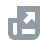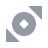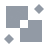Pay with
Markets
Derivatives
Earn
Finance
NFT
Institutional
Feed
USD

# FAQAccount FunctionsTutorialBinance Fan TokenBinance EarnCrypto Deposit/WithdrawalCrypto DerivativesFinanceAPISecurityOther TopicsBinance ConvertNFTVIP
Home
Support Center
FAQ
Tutorial
BNB Balance Calculation

# BNB Balance Calculation

2021-04-19 06:18
Binance calculates your daily BNB balance using the Daily Average BNB Balance of your account, including BNB held in normal Binance Accounts, Margin Accounts, Binance Crypto Loans Collateral, Sub-Accounts, Binance Simple Earn Accounts, BNB Vault Accounts, Futures Wallet Accounts, Binance Funding Wallet Accounts, Launchpool Accounts, and DeFi Staking Accounts.
Total Daily Average BNB Balance = Sum of the daily averages of the following:
• Spot Account Balance
• Margin Account Balance
• Binance Crypto Loans Collateral Balance
• Sub-Accounts Balance
• Simple Earn Account Balance
• BNB Vault Account Balance
• Futures Wallet Balance
• Binance Funding Wallet Balance
• Launchpool Account Balance
• DeFi Staking Account Balance
Note:
• Average Spot Account Balance is calculated using the sum of the hourly snapshots of BNB balance in the spot account in a day divided by 24 (hours).
• Daily Average Sub-Account Balance is calculated as the sum of the hourly snapshots of the BNB balance in the sub-account in a day divided by 24 (hours).
• The sum of the hourly snapshots of the Net BNB balance (Net BNB Balance = BNB Total Balance - Borrowed BNB - BNB Interest) in both master and sub-account margin accounts in a day divided by 24 (hours). Please note that margin account balances may be a negative balance.
• Daily Average Binance Crypto Loans Account BNB Collateral Balance is calculated as the sum of the hourly snapshots of the BNB collateral balance in the Binance Crypto Loans account in a day divided by 24 (hours).
• Daily Average Simple Earn Account Balance is calculated as the sum of the hourly snapshots of the BNB balance in the Binance Simple Earn Account in a day divided by 24 (hours).
• Daily Average BNB Vault Account Balance is calculated as the sum of the hourly snapshots of the BNB balance in the BNB Vault Account in a day divided by 24 (hours).
• Daily Average Binance Futures Wallet Balance is calculated as the sum of the hourly snapshots of the BNB balance in the Binance Futures Account in a day divided by 24 (hours).
• Daily Average Binance Funding Wallet Balance is calculated as the sum of the hourly snapshots of the BNB balance in the Binance Funding Wallet Account in a day divided by 24 (hours).
• Daily Average Binance Launchpool Account Balance is calculated as the sum of the hourly snapshots of the BNB balance in the Binance Launchpool Account in a day divided by 24 (hours).
• Daily Average DeFi Staking Account Balance is calculated as the sum of the hourly snapshots of the BNB balance in the DeFi Staking Account in a day divided by 24 (hours).
• Daily Average Dual Investment BNB Balance is calculated as the sum of the hourly snapshots of the BNB balance in the Dual Investment Account in a day divided by 24 (hours)
• Once your BNB tokens are added into Liquid Swap liquidity pools, your BNB will no longer be counted toward your average BNB calculations for various activities on Binance.